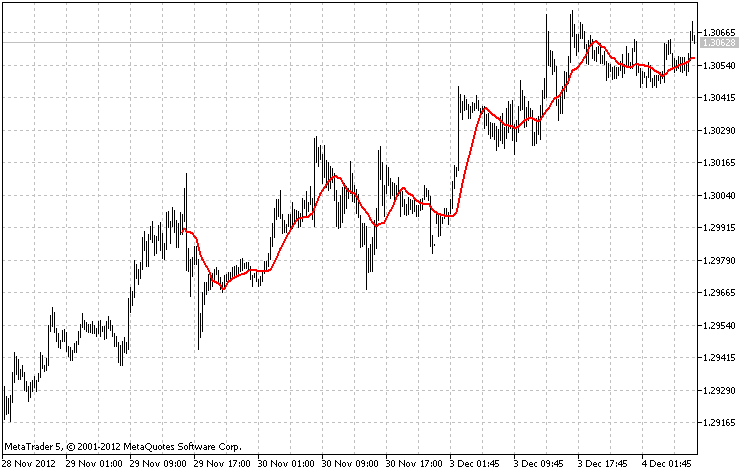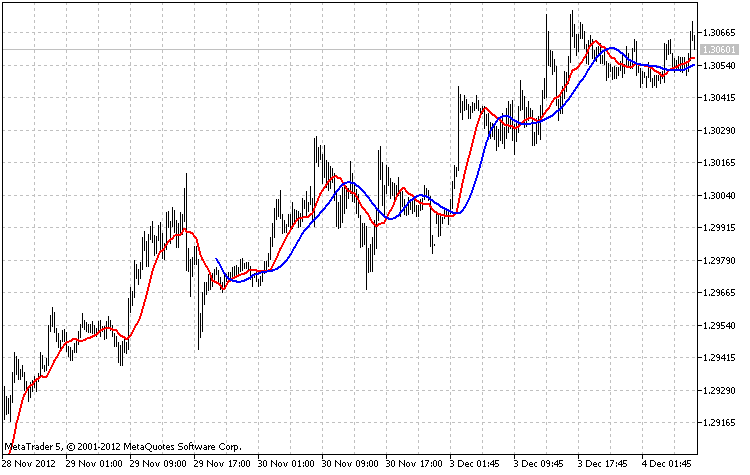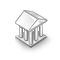Interesting script?
So post a link to it -
let others appraise it

You liked the script? Try it in the MetaTrader 5 terminal# The class for drawing Moving Average using the ring buffer - indicator for MetaTrader 5

Views:
4473
Rating:
Published:
2012.12.27 11:56
Updated:
2016.11.22 07:32

Description

The CMAOnRingBuffer class is designed for calculation of Moving Averages (Moving Average) using the algorithm of the ring buffer

Declaration

`class CMAOnRingBuffer :public CArrayRing`

Title

`#include <IncOnRingBuffer\CMAOnRingBuffer.mqh>`

File of the CMAOnRingBuffer.mqh class should be placed in the IncOnRingBuffer folder that need to be established in MQL5\Include\. Two files with the examples used by the class from this folder are attached to the description. File with the class of the ring buffer also must be in this folder.

Class methods

```//--- initialization method:
bool Init(                                // if error it returns false, if successful - true
int            ma_period   = 14,       // period of Moving Average smoothing
ENUM_MA_METHOD ma_method   = MODE_SMA, // method of Moving Average smoothing
int            size_buffer = 256,      // the ring buffer size, the number of stored data
bool           as_series   = false     // true, if a time series, false if a usual indexing of the input data
);
```
```//--- method of calculation based on a time series or indicator buffer:
int MainOnArray(                  // returns the number of the processed elements of the array
const int     rates_total,     // size of array array[]
const int     prev_calculated, // processed elements of the array in the previous call
const double &array[]          // array for the calculation
);
```
```//--- method of calculation based on separate series elements of the array
double MainOnValue(              // returns MA value for the set element
const int    rates_total,     // the size of the array
const int    prev_calculated, // processed elements in the array
const int    begin,           // from where the significant data of the array starts
const double value,           // the array element value
const int    index            // the array element index
);
```
```//--- methods of access to the data:
int    BarsRequired();   // Returns necessary number of bars to draw the indicator
string Name();           // Returns the indicator name
string MAMethod();       // Returns the method of smoothing in the form of the text line
int    MAPeriod();       // Returns the period of smoothing
```

To get the calculated data of the indicator from the ring buffer is possible as from the usual array. For example:

```#include <IncOnRingBuffer\CMAOnRingBuffer.mqh>
CMAOnRingBuffer ma;
...
//+------------------------------------------------------------------+
//| Custom indicator iteration function                              |
//+------------------------------------------------------------------+
int OnCalculate(const int rates_total,
const int prev_calculated,
const int begin,
const double &price[])
{
//--- calculation of the indicator:
ma.MainOnArray(rates_total,prev_calculated,price);

...

//--- copy data from the "ma" ring buffer to the indicator:
for(int i=start;i<rates_total;i++)
{
MA_Buffer[i]=ma[rates_total-1-i];
}
return(rates_total);
}```

Please note, that indexing in the ring buffer is the same as in a time series.

Examples

1. The indicator calculates the Test_MA_OnArrayRB.mq5 file on the basis of the price time series. The MainOnArray() method application is demonstrated
2. The Test_MA_OnValueRB.mq5 file demonstates use of the MainOnValue() method. At first the MA indicator is calculated and draws. Then on the basis of the ring buffer of this indicator, one more МА indicator is calculated.The result of the work of the Test_MA_OnArrayRB.mq5 with the size of the ring buffer of 256 elementsThe result of the work of the Test_MA_OnValueRB.mq5 with the size of the ring buffer of 256 elements

When writing code the developments of MetaQuotes Software Corp.Integer and GODZILLA were used.

Translated from Russian by MetaQuotes Software Corp.
Original code: https://www.mql5.com/ru/code/1342The class to create the ring buffer

The class allows to organize the mini time series, indicator minibuffers, short sized buffers to store intermediate stream data inside the Expert Advisor or indicator.Exp_ColorLeManTrend

The Exp_ColorLeManTrend trading system is based on changes of the trend direction displayed by the ColorLeManTrend indicatorCombinatorics

Initial library of combinatorics functions.Exp_ColorTrend_CF

The Exp_ColorTrend_CF trading system is based on change of the trend direction displayed by the ColorTrend_CF indicator